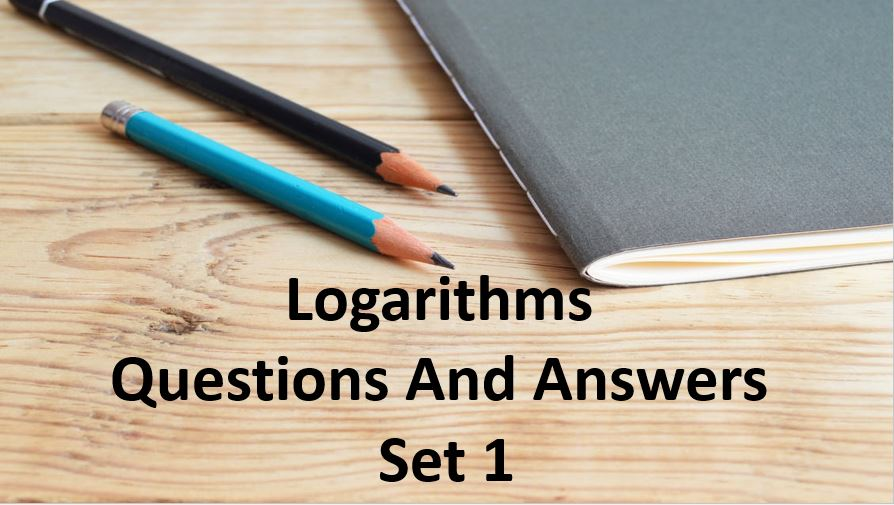Monday, September 27, 2021
Home > Question and Answer > Logarithm Question And Answer Set 1

# Logarithm Question And Answer Set 1# Logarithm Questions and Answer Set 1

Hi students, welcome to Amans Maths Blogs (AMB). On this post, you will get the Logarithm Questions and Answer Set 1 is the collection of some important questions. Practice these questions for SSC CGL CHSL CAT NTSE NSEJS exams etc. It will help you to practice the questions on the topics of maths as logarithm based questions of algebra.

Logarithm Questions and Answer Set 1: Ques No 1

If log5 25 + log5 5 = x, then the value of x

Options:

A. 3

B. 5

C. 7

D. 8

Logarithm Questions and Answer Set 1: Ques No 2

If 2 log 2 + log a + log b = 2 log (a + b), then which of the following is true?

Options:

A. a + b = 0

B. a – b = 0

C. a/b = 2

D. ab = 4

Logarithm Questions and Answer Set 1: Ques No 3

The least value of the expression 2 log10(x) – logx(1/100) for x > 1 is

Options:

A. 2

B. 3

C. 6

D. 4

Logarithm Questions and Answer Set 1: Ques No 4

If logx(1/8) = -3/4, then the value of x is

Options:

A. 24

B. 8

C. 16

D. 4

Logarithm Questions and Answer Set 1: Ques No 5

If log5(3) + log5(3x + 1) = 1 + log5(x + 3), then the value of x is

Options:

A. 3

B. 4

C. 2

D. 6

Logarithm Questions and Answer Set 1: Ques No 6

If loga(x2 – 10) – loga(x) = 2loga(3), then the value of x is

Options:

A. -1

B. 2

C. 10

D. 4

Logarithm Questions and Answer Set 1: Ques No 7

If log10(2x + x – 41) = x(1 – log105), then the value of x is

Options:

A. 6

B. 5

C. 40

D. 41

Logarithm Questions and Answer Set 1: Ques No 8

The value of log2 log2 loglog3 273 is

Options:

A. 0

B. 1

C. 2

D. 3

Logarithm Questions and Answer Set 1: Ques No 9

If log(a/b) + log(b/a) = log(a + b), then

Options:

A. a + b = 1

B. a – b = 1

C. a = b

D. a2 – b2 = 1

Logarithm Questions and Answer Set 1: Ques No 10

If log 2 = x, log 3 = y and log 7 = z, then the value of log () in terms of x, y and z is

Options:

A. 4x + 2y/3 + z/3

B. 2x + y/3 + z/3

C. 3x + 2y/3 + z/3

D. x + 2y/3 + z/3